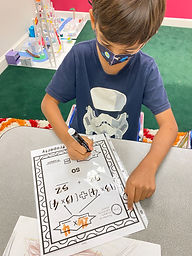Ms. Kylie

Target 1​

Lesson Type:

Continuation

Geometry

:

Shape

Measure, draw, and classify angles.

1:

Accurately measure angles.

2:

Understand that angles have types based on their degree measures (acute, obtuse, and right).

3:

Understand that angles can be composed or decomposed, using addition and subtraction.

4th

Vocabulary:

Acute Angle, Obtuse Angle, Right Angle, and Straight Angle, Vertex

Activities:

-Students reviewed measuring angles with a protractor. Then they drew angles and exchanged papers to measure each other's angles.

-Students composed angles by adding the degrees of each angle together. They also decomposed angles by subtracting total measurement of two angles and the given degrees of one angle.

-Students designed and drew a town using a protractor to measure angles of each place they included in their town.Home Exploration

Guiding Questions:Absent Students:

Target 2

:

1:

Identify the Distributive Property of Multiplication.

2:

Understand that the Distributive Property of Multiplication allows for numbers to be separated into parts (multiply a sum by multiplying each addend separately and then adding the products).

4th

Vocabulary:

Distributive Property

Activities:

-Students were given a double digit number multiplied by a single digit number and used the distributive property of multiplication to solve the problem.

-Students learned multiplying two factors together gives the same result as breaking one factor (double digit number) into two addends and multiplying it each addend with the other factor and then adding the two products together.Home Exploration

Guiding Questions:Target 3

:

Vocabulary:

Activities:Home Exploration